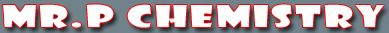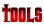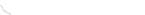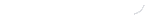activity seriesbond energybond lengthscalculatorcommon namesconversion factors: energyconversion factors: lengthconversion factors: massconversion factors: pressureconversion factors: temp.conversion factors: volumecovalent prefixesdensity solverdiatomic elementse-config. chartelement listgas law formulasKa's of polyprotic acidsKa's of weak acidsKb's of weak basesmetric conversion chartmole conversion chartmolecular geometriesorganic prefixesperiodic tableperiodic table (flash)pH/pOH converterpolyatomic ionspressure converterSI unitssolubility chartsolubility of salts rulessolubility product constantsstoichiometry charttemp. conversiontemp. formulasthermodynamic datavapor pressure of water
Metric Conversion

All measurements in chemistry are made using the metric system. In using the metric system often times you will have to convert between one unit and another. The steps below show you how to convert between units using the label factor method. There are other methods for converting between units. I prefer this method because it will be used for other calculations later in the year. For metric conversions use the chart below.

 Metric Conversions symbol prefix value conversion factor G giga- 109 10-9 M mega- 106 10-6 K kilo- 103 10-3 base units (gram/meter/liter) 100 or 1 100 or 1 d deci- 10-1 101 c centi- 10-2 102 m milli- 10-3 103 µ micro- 10-6 106 n nano- 10-9 109 p pico- 10-12 1012

Note the difference between the two columns on the right side of the chart below. The column labeled value is the actual value of the metric unit. The column labeled conversion factor is used only when converting between metric units. Please see that the power of ten values in the conversion factor column have the opposite sign of the power of ten values in the value column.

Example: Convert 18.3 kilograms to centigrams.

Step 1. Write the given value and unit over 1.

18.3 kilograms
.........1

Step 2. Write a multiplication sign . In this step, the numerator (on top) is the conversion factor and unit to which you are converting. The denominator (on the bottom) is the conversion factor and unit of the given number. Your problem should be set up such that the given unit cancels out.

18.3 kilograms x 102 centigrams =
.........1.............. 10-3 kilograms

Step 3. Calculate the problem. Remember when dividing powers of 10, subtract the denominator from the numerator.

18.3 kilograms x 102 centigrams = 18.3 x 105
.........1.............. 10-3 kilograms
* (I got 105 by subtracting, 2 - (-3) = 5 )

Step 4. Put your answer in proper scientific notation. 1.83 x 106 centigrams

 You may also find these related tutorials helpfulCompounds in Crust of EarthDensityElements in Crust of EarthHow To Round NumbersMetric ConversionScientific NotationSI UnitsSignificant Figure CalculationsSignificant Figures